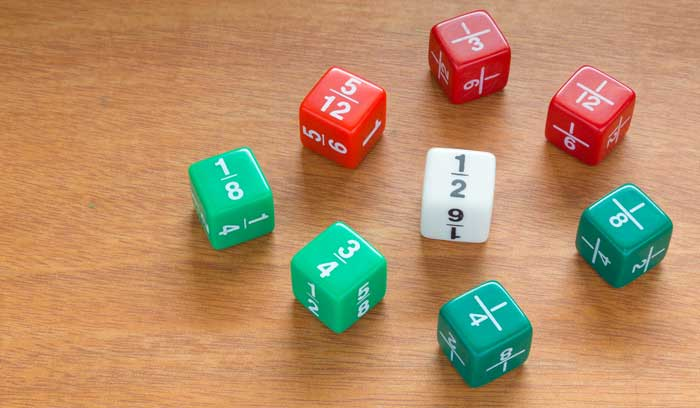Calc

# Fractions Calculator

## Standard fractions calculator

Use this calculator to add, subtract, multiply and divide basic fractions.

## Mixed fractions calculator

Calculate using mixed fractions. For simple fractions, enter the fraction with a slash (/). Example: 3/4. For mixed numbers, use spaces. Example: 16 3/4.

## Simplify a fraction

Find the common factor of a fraction and then simplify it to its lowest form. A decimal conversion is also provided.

Use this popular fractions calculator to add, subtract, multiply and divide fractions quickly and easily, including mixed number fractions. The calculator gives explanation of the working steps involved and simplifies the result using the greatest common denominator.

Should you wish to convert a decimal number to a fraction, give the decimal to fraction calculator a try.

#### Disclaimer

Whilst every effort has been made in building this fractions calculator, we are not to be held liable for any special, incidental, indirect or consequential damages or monetary losses of any kind arising out of or in connection with the use of the converter tools and information derived from the web site. This fractions calculator is here purely as a service to you, please use it at your own risk. Do not use calculations for anything where loss of life, money, property, etc could result from inaccurate conversions.## Fractions calculator - FAQ

I've been asked to provide some information about how the fractions calculator works out its results. So, I've included the formulae below along with some examples. Note that you need to have JavaScript enabled in your browser for these fraction formulae and examples to appear correctly formatted.In order to add fractions together, use the following formula:

$$\dfrac{a}{b} + \dfrac{c}{d} = \dfrac{ad + bc}{bd}$$

Example of how to add fractions:

$$\dfrac{2}{3} + \dfrac{1}{4} = \dfrac{(2\times4) + (3\times1)}{3\times4} = \dfrac{11}{12}$$

### Subtracting fractions

To subtract fractions, the following formula can be used:

$$\dfrac{a}{b} - \dfrac{c}{d} = \dfrac{ad - bc}{bd}$$

Example of how to subtract fractions:

$$\dfrac{2}{3} - \dfrac{1}{4} = \dfrac{(2\times4) - (3\times1)}{3\times4} = \dfrac{5}{12}$$

### Multiplying fractions

When multiplying fractions, this formula will help:

$$\dfrac{a}{b} \times \dfrac{c}{d} = \dfrac{ac}{bd}$$

Example of how to multiply fractions:

$$\dfrac{2}{3} \times \dfrac{1}{4} = \dfrac{(2\times1)}{(3\times4)} = \dfrac{2}{12} = \dfrac{1}{6}$$

### Dividing fractions

If you are looking to divide two fractions, give this formula a try:

$$\dfrac{a}{b} \div \dfrac{c}{d} = \dfrac{ad}{bc}$$

Example of how to divide fractions:

$$\dfrac{2}{3} \div \dfrac{1}{4} = \dfrac{(2\times4)}{(3\times1)} = \dfrac{8}{3}$$

To learn how to calculate fractions using these formulae, take a look at our article how to add, subtract, multiply and divide fractions. If you would like help with converting between decimals and fractions, see our article how to convert a decimal to a fraction.

When it comes to performing a mathematical calculation, it is important to carry out the operations in the correct order. This is where the order of operations comes in. Thankfully, there are a couple of mnemonics to assist us with remembering the order of operations. Read our articles about PEMDAS (US) and BODMAS (UK).